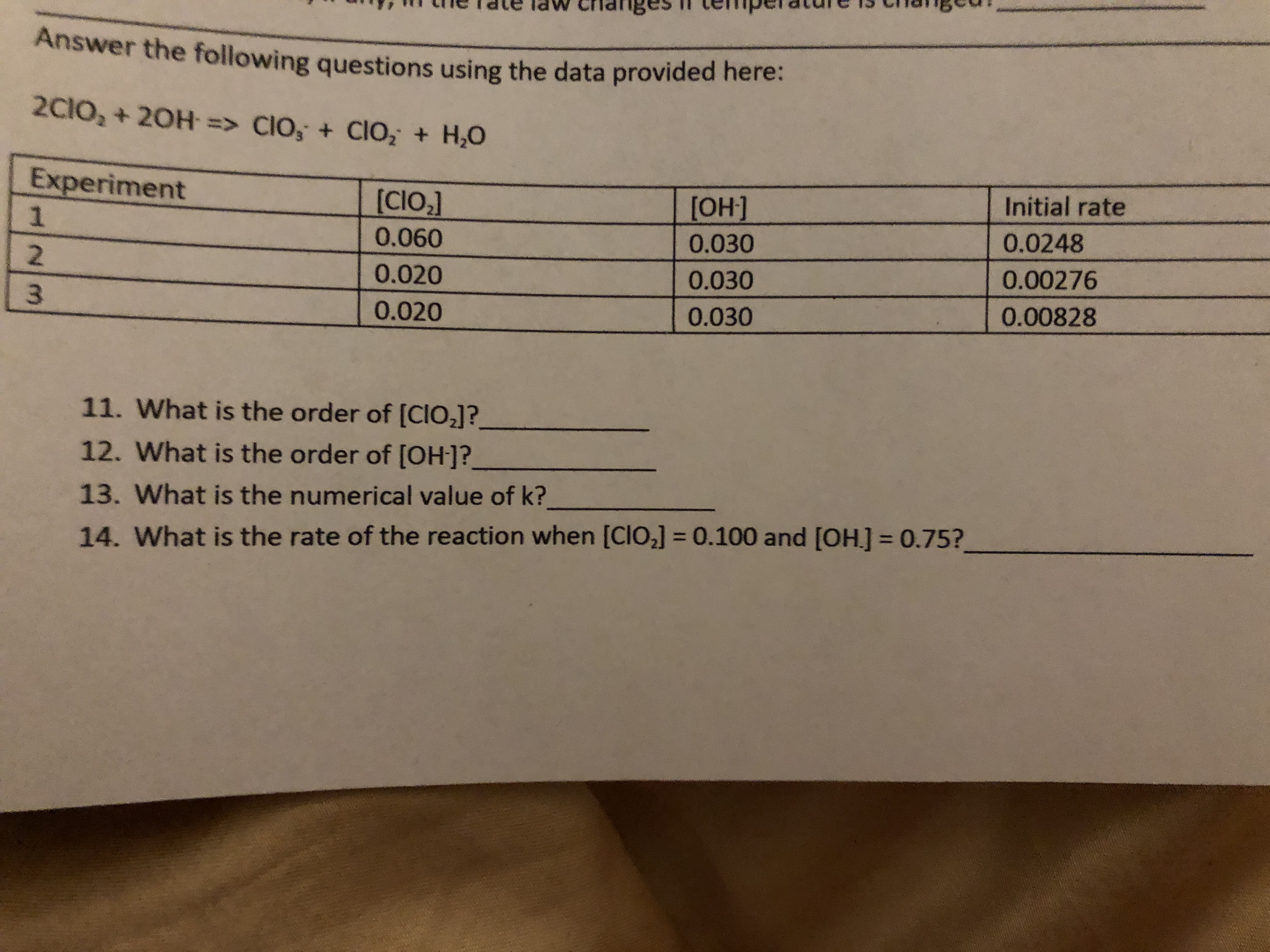# HINM15 Chageu!meiadua II saaupua MPI anPdldte lcrhangesAnswer the following questions using the data provided here:2CIO2 + 20H > Clo, + ClO, +H,0Experiment[CIO21[OH]Initial rate10.02480.0600.03020.0200.0300.0027630.0200.0300.0082811. What is the order of [CIO2]?12. What is the order of [OH]?13. What is the numerical value of k?14. What is the rate of the reaction when [CIO] = 0.100 and [OH] = 0.75?

Question
4 viewshelp_outlineImage TranscriptioncloseHINM 15 Chageu! meiadua II saaupua MPI anP dl dte l crhanges Answer the following questions using the data provided here: 2CIO2 + 20H > Clo, + ClO, +H,0 Experiment [CIO21 [OH] Initial rate 1 0.0248 0.060 0.030 2 0.020 0.030 0.00276 3 0.020 0.030 0.00828 11. What is the order of [CIO2]? 12. What is the order of [OH]? 13. What is the numerical value of k? 14. What is the rate of the reaction when [CIO] = 0.100 and [OH] = 0.75? fullscreen
check_circle

Step 1

Hey, since there are multiple questions posted, we will answer first question. If you want any specific question to be answered then please submit that question only or specify the question number in your message.

Step 2

For the given reaction, rate of reaction is given by equation 1.

Rate = k [ClO2]m[OH-]n            (1)

k is...

### Want to see the full answer?

See Solution

#### Want to see this answer and more?

Solutions are written by subject experts who are available 24/7. Questions are typically answered within 1 hour.*

See Solution
*Response times may vary by subject and question.
Tagged in

### Chemical Kinetics